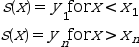Scilab Home page | Wiki | Bug tracker | Forge | Mailing list archives | ATOMS | File exchange
Change language to: English - Français - Português - 日本語 -

See the recommended documentation of this function

Scilab help >> Interpolation > interp

# interp

cubic spline evaluation function

### Calling Sequence

`[yp [,yp1 [,yp2 [,yp3]]]]=interp(xp, x, y, d [, out_mode])`

### Arguments

xp

real vector or matrix

x,y,d

real vectors of the same size defining a cubic spline or sub-spline function (called `s` in the following)

out_mode

(optional) string defining the evaluation of `s` outside the [x1,xn] interval

yp

vector or matrix of same size than `xp`, elementwise evaluation of `s` on `xp` (yp(i)=s(xp(i) or yp(i,j)=s(xp(i,j))

yp1, yp2, yp3

vectors (or matrices) of same size than `xp`, elementwise evaluation of the successive derivatives of `s` on `xp`

### Description

Given three vectors `(x,y,d)` defining a spline or sub-spline function (see splin) with `yi=s(xi), di = s'(xi)` this function evaluates `s` (and `s', s'', s'''` if needed) at `xp(i)` :The `out_mode` parameter set the evaluation rule for extrapolation, i.e. for `xp(i)` not in [x1,xn] :

"by_zero"

an extrapolation by zero is done

"by_nan"

extrapolation by Nan

"C0"

the extrapolation is defined as follows :"natural"

the extrapolation is defined as follows (p_i being the polynomial defining `s` on [x_i,x_{i+1}]) :"linear"

the extrapolation is defined as follows :"periodic"

`s` is extended by periodicity.

### Examples

```// see the examples of splin and lsq_splin

// an example showing C2 and C1 continuity of spline and subspline
a = -8; b = 8;
x = linspace(a,b,20)';
y = sinc(x);
dk = splin(x,y);  // not_a_knot
df = splin(x,y, "fast");
xx = linspace(a,b,800)';
[yyk, yy1k, yy2k] = interp(xx, x, y, dk);
[yyf, yy1f, yy2f] = interp(xx, x, y, df);
clf()
subplot(3,1,1)
plot2d(xx, [yyk yyf])
plot2d(x, y, style=-9)
legends(["not_a_knot spline","fast sub-spline","interpolation points"],...
[1 2 -9], "ur",%f)
xtitle("spline interpolation")
subplot(3,1,2)
plot2d(xx, [yy1k yy1f])
legends(["not_a_knot spline","fast sub-spline"], [1 2], "ur",%f)
xtitle("spline interpolation (derivatives)")
subplot(3,1,3)
plot2d(xx, [yy2k yy2f])
legends(["not_a_knot spline","fast sub-spline"], [1 2], "lr",%f)
xtitle("spline interpolation (second derivatives)")``````// here is an example showing the different extrapolation possibilities
x = linspace(0,1,11)';
y = cosh(x-0.5);
d = splin(x,y);
xx = linspace(-0.5,1.5,401)';
yy0 = interp(xx,x,y,d,"C0");
yy1 = interp(xx,x,y,d,"linear");
yy2 = interp(xx,x,y,d,"natural");
yy3 = interp(xx,x,y,d,"periodic");
clf()
plot2d(xx,[yy0 yy1 yy2 yy3],style=2:5,frameflag=2,leg="C0@linear@natural@periodic")
xtitle(" different way to evaluate a spline outside its domain")```• splin — cubic spline interpolation
• lsq_splin — weighted least squares cubic spline fitting

### History

 Версия Описание 5.4.0 previously, imaginary part of input arguments were implicitly ignored.